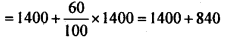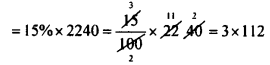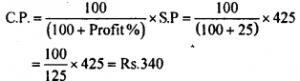# KSEEB Solutions for Class 8 Maths Chapter 9 Commercial Arithmetic Ex 9.3

In this chapter, we provide KSEEB SSLC Class 8 Maths Chapter 9 Commercial Arithmetic Ex 9.3 for English medium students, Which will very helpful for every student in their exams. Students can download the latest KSEEB SSLC Class 8 Maths Chapter 9 Commercial Arithmetic Ex 9.3 pdf, free KSEEB SSLC Class 8 Maths Chapter 9 Commercial Arithmetic Ex 9.3 pdf download. Now you will get step by step solution to each question.

### Karnataka State Syllabus Class 8 Maths Chapter 9 Commercial Arithmetic Ex 9.3

Question 1.
An article marked Rs. 800 is sold for Rs. 704. Find the discount and discount percent.
M.P = Rs. 800.
S.P = Rs. 704
Discount = MP – S.P = 800 – 704 = Rs. 96
Discount percentage =$\frac { Discount }{ M.P }$ × 100$\frac { 90 }{ 800 }$ × 100 = 12%

Question 2.
A dress is sold at Rs. 550 after allowing a discount of 12%. Find its marked price.
S.P. = Rs. 550
Rate of discount = 12%
Let the marked price be Rs. 100.
Then S.P = 100 – 12 = 88
If S.P. isRs. 88 then the marked price is Rs.100
If the S.P. is Rs. 550 the M.P. is$\frac{100 \times 550}{88}=\frac{55000}{88}$ = 625
Marked price = Rs. 625.

Question 3.
A shop keeper buys a suit piece for Rs. 1400 and marks it 60% above the cost price. He allows a discount of 15% on it. Find the marked price of the suit piece and also the discount is given.
C.P = Rs. 1400.
M.P = 60% above the C.P.
M.P = 1400 + 60% of 1400M.P= Rs. 2240
Discount = Rate of discount × M.P.Discount = Rs. 336.

Question 4.
A dealer marks his goods 40% above the cost price and allows a discount of 10%. Find the profit percent.
Let the cost price be Rs. 100.
Then marked price is 100 + 40 = Rs. 140.
Rate of discount = 10%
If the marked price is Rs.100 the S.P. is 100 – 10 = Rs. 90
If the marked price is Rs. 140 the S.P.∴ C.P. = Rs. 100
S.P. = Rs. 126
S.P > C.P
∴ There is profit Profit = 126 – 100 = Rs. 26
Profit percentage = 26% [∴ C.P. is 100]

Question 5.
A dealer is selling an article at a dis¬count of 15%. Find
(i) The selling price if the market price is Rs. 500
(ii) The cost price if he marks a 25% profit.
Rate of discount is 15%
(i) If the M.P. is Rs. 100 the S.P. is 100 – 15 = Rs. 85 So if the M.P. is 500 the S.P. is
85100 × 500
S.P=Rs.425
(ii) S.P. = Rs. 425
Profit % = 25%The cost price is Rs.340.

All Chapter KSEEB Solutions For Class 8 maths

—————————————————————————–

All Subject KSEEB Solutions For Class 8

*************************************************

I think you got complete solutions for this chapter. If You have any queries regarding this chapter, please comment on the below section our subject teacher will answer you. We tried our best to give complete solutions so you got good marks in your exam.

If these solutions have helped you, you can also share kseebsolutionsfor.com to your friends.

Best of Luck!!